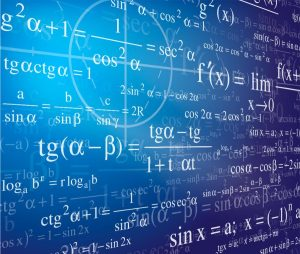## What Is Delta In Math?

### What Is Delta In Math?

In mathematics, the delta symbol means “change” or “the change.” It can also refer to the rate of change. In addition, it can be used in word problems and equations to show a change in one variable.Lower-case delta (d) may represent an angle in any geometric shape, primarily because geometry has its roots in the work of Euclid in ancient Greece. Consequently, mathematicians marked their angles with Greek letters.

The delta symbol is often used in the fields of physics, chemistry, and engineering. It is also a commonly used mathematical symbol in word problems and equations.

In algebra, upper-case delta (D) typically represents the discriminant of a polynomial equation, especially the quadratic. For example, if the quadratic ax2 + bx =c was given, D would equal b2 – 4ac, and it should look like this:

A lower-case delta (d) indicates partial derivatives; it differs from regular derivatives because the function has multiple variables but only one is considered: the other variables stay fixed. For example, the partial derivative of function f looks like this: df over dx.

### Using the Delta Math app

The mission of DeltaMath is to provide students with an innovative approach to learning and checking math answers. Teachers can mix and match problem-sets, control rigor and variety, and track student progress with a powerful, easy-to-use app. Plus, students get access to help videos.